Courses

# Types of Electrolytes, Acids, Bases and Salts Class 11 Notes | EduRev

## Class 11 : Types of Electrolytes, Acids, Bases and Salts Class 11 Notes | EduRev

The document Types of Electrolytes, Acids, Bases and Salts Class 11 Notes | EduRev is a part of the Class 11 Course Chemistry Class 11.
All you need of Class 11 at this link: Class 11

The equilibrium established between the unionised molecules and the ions in the solution of weak electrolytes is called ionic equilibrium.

e.g.,

CH3 COOH ⇔ CH3COO + H

Electrolytes:

Chemrcal substances which can ccnduct electricity in their aqueous stare or tn molten state are called electrolytes. The conduction of current through electrolyte is due to the movement of ions.

1. Strong Electrolytes

Electrolytes which dissociate almost completely into constituent ions in aqueous solution are known as strong electrolytes. e.g.. all salts (except HgCl2, CdBr2)’ mineral acids like HCl, H2SO4, HNO3 etc.. and bases like NaOH. KOH. etc.

2. Weak Electrolytes

Electrolytes which dissociate to a lesser extent in aqueous solution are called weak electrolyte. All organic acids (except sulphonic acids), and bases like NH3. NH4OH, amines etc.

Degree of Ionisation or Degree of Dissociation (α):

It is the fraction of the total number of molecules which ionise (dissociate)into constituent ions.

α = (number of molecules ionised or dissociated/total number of molecules taken)

For strong electrolytes,

α = 1

For weak electrolytes

α < 1

Values of the degree of dissociation (ex)depends upon the following factors

1. nature of solute
2. nature of solvent
3. concentration
4. temperature

Ostwald’s Dilution Law:

According to Ostwald. the degree of dissociation (ex)of weak electrolyte is inversely proportional to the square root of the molar concentration of the solution.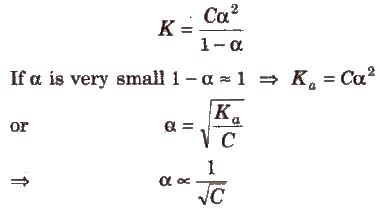Hore K is dissociation constant and C is molar concentration of the solution.

Acids and Bases

Earlier Definitions of Acids and Bases

Earlier definitions of acids and bases was given by Robert Boyle, who classified them on the basis of their properties. According to him, acids are the substance which have sour taste. turns blue litmus red. liberate hydrogen with metals, conduct electricity in aqueous solution and neutralise bases.

Bases are the substance which have bitter taste, turns red litmus blue, soapy to touch, conduct electricity in aqueous solution and neutralise acids.

Arrhenius Concept of Acids and Bases

• Acid is a chemical substance which dissociates in aqueous solution to give hydrogen ions (H+) or hydronium ions (H3O+).
• Base is a chemical substance which dissociates in aqueous solution to give hydroxyl ions (OH).
• Arrhenius theory fails to explain the acidic and basic behaviour in non-aqueous solutions. It cannot explain the acidic character of A1Cla. BFa and basic character of NH3, PH3, etc.

Bronsted Concept of Acids and Bases

Acid is a chemical substance that can donate a proton (H+) to some other substance and a base is a chemical substance that can accept a proton from other substance. Thus, an acid is a proton donor (protongenic) and a base is proton acceptor (protopbilic).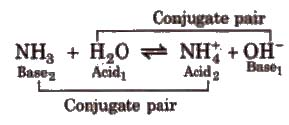Strong acid has weak conjugate base and weak acid has strong conjugate basco Strong base has weak conjugate acid and weak base has strong conjugate acid.

HClO4 is the strongest while HCN is the weakest hydracid known.

CsOH is the strongest base known.

Amphoteric or arnphiprotic substance or ampholytes are the substance which act as an acid as well as a base, e.g.• water acts as an acid with NH3 and a base with acetic acid.

The order of acidic strength of some acids is

HClO4 > HBr> H2SO4 > HCl> HNO3

Greater the Ka value of an acid (or lesser the pKa), stronger is the acid. Similarly. greater the Kb (or lesser the pKb) of a base. stronger is the base.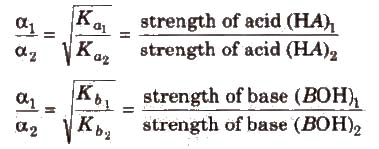Leveling Effect

The adds like HClO4 H2SO4, HNO3 etc. react with water almost completely to form H3O+ions. Therefore, all the strong acids in aqueous solutionsappear equally strong and their relative strengths in aqueous solution cannot be compared. Since H3O+ is the strongest acid in water. the strength of above acids come down to the level of H3O+ strength in water. Similarly.strong bases like NaOH. KOH. Ba(OH)2 come down to the strength of OH ion in water.

This is called levling effect.

Lewis Concept of Acids and Bases

Lewis acid is a chemical substance which can accept a pair of electrons,

e.g.,

1. Molecules with incomplete octet of central atom like AlCl3 ,BeCl2, MgCl2, etc.
2.  Simple cations like Ag+, Na+, etc.
3. Molecules in which the central atom has vacant d-orbital, e.g.,SF4, SnC14 PF3 etc.

Lewis base is a chemical substance which can donate a pair of electrons. e.g.,

1. Neutral molecules containing lone pairs like NH3, RNH2, ROH etc.
2. Negatively charged species like CN, Cl. OH, etc.
3. In coordination complexes, the ligands act as Lewis base.

Limitations of Lewis Concept

1. It does not explain the behaviour of protonic acids such as HCl, H4SO4, HNO3 etc.
2. It does not predict the magnitude of relative strength of acids and bases.

All Bronsted-Lowry’s acids are Lewis acids while acids need not be Bronsted-Lowry’s acids.

The Ionization Constant of Water Ionic product is the product of the concentration of hydronium ions and hydroxyl ion in pure water, which remains constant at a particular temperature. It is symbolized by Kw. At 298 K, ionic product of water (KW) is given as KW: = [H3O+] [OH] = 1 x 10-14 mol2L.

The value of Kw increases with increase in temperature.

The pH Scale

pH is defined as the negative logarithm of hydrogen ion concentration.

pH = – log [H+] and [H+] = lO-pH

Total [H+] or [OH] in a mixture of two strong acids or bases = (ΣNV/ΣV)

Similarly, negative logarithm of hydroxyl ion concentration is pOH.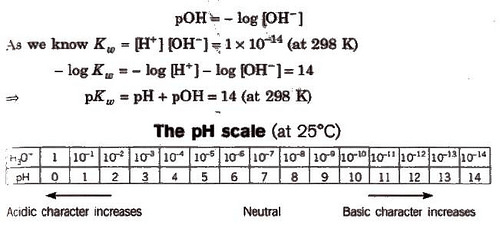pH value of an acid having H+ concentration less than 10-7, is always in between 6 and 7. For 10-8 N HCl solution. it is 6.958.Similarly, for 10-8 NaOH solution, the pH is 7.04 (because basic solutions always have pH 77.)

pH of solution is accurately measured by pH meter or emf method or roughly by pH paper or indicator paper.

(PH can be zero in 1 N Hel solution or it can be negative for more. concentrated solution like 2N, 3N, lON, etc,

pH range for some important substances are :

Gastric juice = 1 – 3
Vinegar = 2.4 – 3.4
Tears – 7.4
Human urine – 4.8 – 8.4
Blood plasma – 7.3 – 7.4
Boil water – 6.5625

Dissociation Constant of Weak

Acid and Weak Base

Let us consider the dissociation of weak acid (HA) as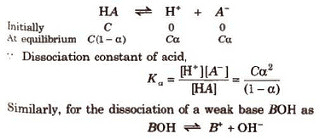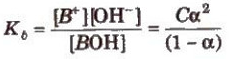Dissociation constant for polyprotic acids and bases. For a tribasic acid,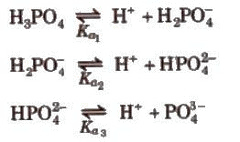The overall dissociation constant (K) is given as

K = K1 x K2 x K3

where, K1 x K2 x K3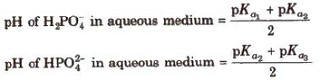Similarly, for a dibasic acid like H2CO3

pH = pka1 + pka2/2)

Buffer Solution

Solution which resists the change in its pH value by addition of a small amount of acid or a base, is called buffer solution.

• Acidic buffer They have pH value < 7, e.g., CH3COOH/CH3COONa, bone acid/borax.
• Basic buffer They have pH value> 7 e.g., NH4OH/NH34Cl

Buffer system present in blood is H2CO3 + NaHCO3.

Henderson-Hesselbalch Equation

Equation used to calculate the pH of a buffer solution.

(i) For acidic buffer,

pH = pKa + log[salt/acid]

(i) pOH = pKb + log[salt/acid]

and pH = 14 – pOH

Here, pKa = -log Ka, pKb = -log Kb and Ka and Kb are dissociation constants of acid and base.

[salt), [acid] and [base) represent molar concentrations of salt, acid and base respectively.

If addition of a strong acid or base changes the pH of a buffer by/unit, the buffer solution is assumed to destroyed, i.e.,

New pH = pKo ± 1

This means the ratio.

[salt]/[acid) or [salt]/[base] = 10 or (1/10)

Buffer Capacity

It is defined as the number of moles of acid or base added in 1 L of solution Lochange the pH by unity.

Buffer capacity (φ) = (no. of moles of acids or base added to 1 L of buffer/change in pH)

Salts

These are the product of reaction between an acid and a base.
This reaction is called neutralisation reaction.

Types of Salts

(a) Normal salts These are obtained by complete neutralisation of an acid with a base, e.g., NaCI, K2SO4, etc.

(b) Acidic salts These are formed by incomplete neutralisation of polybasic acids. e.g., NaHCO3, Na2SO4 etc.

(c) Basic salts These are formed by incomplete neutralization of polyacidic base, e.g., Mg(OH)Cl, Bi(OH)2Cl, etc.

(d) Double salts These are formed by the combination of two simple salts and exist only in solid state, e.g., Mohr salt or ferrous ammonium sulphate (FeSO4.(NH4)2SO4.6H2O], alum, etc.

(e) Complex salts These are formed by the combination of simple salts or molecular compounds. These are stable in solid state well as in solutions.

The properties of their solutions are different from the properties of substances from which they have been constituted.

(f) Mixed salts These salts furnish more than one cation or more than one anion when dissolved in water, e.g., Ca(OCl)Cl, NaKSO4, etc.

Offer running on EduRev: Apply code STAYHOME200 to get INR 200 off on our premium plan EduRev Infinity!

## Chemistry Class 11

136 videos|245 docs|180 tests

,

,

,

,

,

,

,

,

,

,

,

,

,

,

,

,

,

,

,

,

,

,

,

,

,

,

,

;